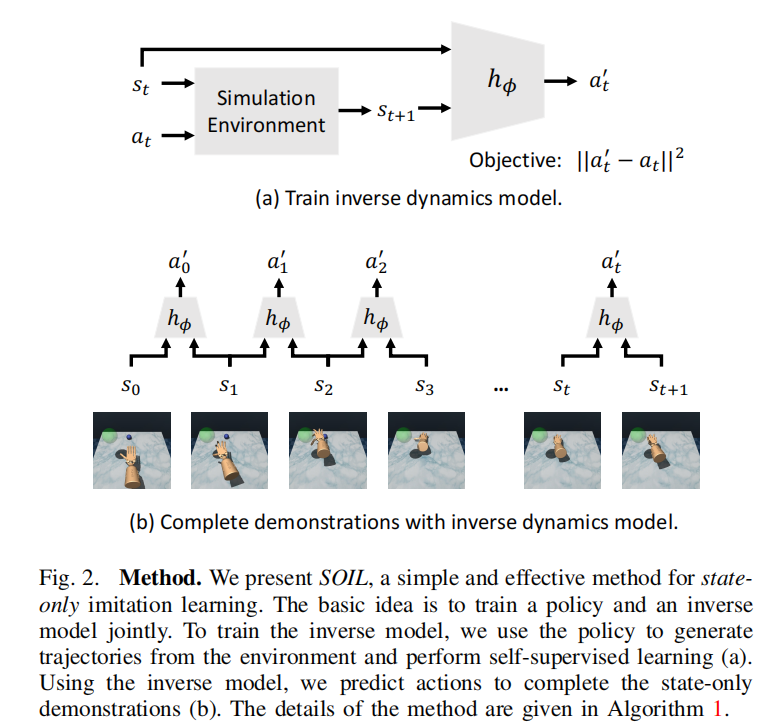# (IROS2021)SOIL

​ 因为灵巧手的操作的domain相对复杂，所以需要引入专家示教的方式要诱导分布。但是通常情况下，在现实世界中，要得到state-action pair是比较困难的，比如从视频中学习。所以这篇文章就训练了一个inverse dynamics model（反向动态模型）来从状态转移中预测对应的动作。

​ 首先，传统的逆向动力学(Robotic Inverse Dynamics)的概念为：已知某一时刻机器人各关节的位置$q$，关节速度$\dot{q}$以及关节加速度$\ddot{q}$，求此时施加在机器人各杆件上的驱动力（力矩）$\tau$。

​ 而在模仿学习中，反向动态模型(Inverse Dynamics model)指的是从状态转移$\{S_t,S_{t+1}\}$到动作$A_t$的映射。

​ 论文给了RL和模仿学习的定义，此处再回顾一下：

### 普通策略梯度算法

$$g=\sum_{(s,a)\in\pi}\nabla_{\theta}\log\pi_{\theta}(a|s)A^{\pi}(s,a)$$

​ 其中的$A^{\pi}(s,a)$是advantage function。

### DAPG算法

​ 我们可以使用示教的方式来加速收敛。示教以state-action pair的形式输入，可以使用DAPG（demo augmented policy gradient）算法。DAPG把原先的策略梯度上添加了一项辅助的模仿项（auxiliary imitation form），也就是：
$$g_{dapg}=g+\lambda_0\lambda_1^k\sum_{(s,a)\in D}\nabla_{\theta}\log\pi_{\theta}(a|s)$$
​ 其中D是state-action示教，而$\lambda_0$和$\lambda_1$是尺度化的超参数来控制模仿学习目标的相对贡献。

## Problem

​ 我们假设模型可以直接访问到灵巧手的关节角度、对应的力、关节的速度。我们也会提供物体的位置。这允许我们单纯地研究state-only imitation learning，而不去纠结一些state estimation的内容。​ (a)图就是反向动态模型的结构，也就是从仿真器中收集到$(s_{t}, s_{t+1}, a_{t})$三元组作为GT，训练一个输入两个state，预测$a_t’$的模型。(b)图就是在说，我们有了反向动力学模型后，只需要在示教数据集上做一遍inference就可以得到state-action demonstration，放到DPAG中进行训练。

​ 所以为了训练反向动态模型，我们需要在仿真器中收集足够多的$(s_{t}, s_{t+1}, a_{t})$来训练，但是如果我们在仿真器中使用初始策略随机采集轨迹的话，由于action空间是非常大的，所以效果会很差。我们需要有一个办法来探索action空间，使其往高目标价值函数方向优化。这就是为什么我们在SOIL算法中每一次都是用当前策略$\pi_{\theta}$下收集的轨迹来训练我们的反向动态模型。因为我们认为专家示教是相对于一系列高reward function的action。迭代可以保证策略逐步收敛，也就是我们的$\pi_{\theta}$正在逐渐接近我们的示教策略。这样的话，对示教动作的估计会越来越精准。

Algorithm: State-Only Imitation Learning(SOIL)
$\textbf{Input:}$ Inverse model $h$, Policy $\pi$, Replay buffer $R$, State-Only Demonstration D.
$\textbf{Initialize:}$ Learnable parameters $\phi$ for $h_{\phi}$, $\theta$ for $\pi_{\theta}$.
$\textbf{for}$ i = 1,2,…,$N_{iter}$ $\textbf{do}$
$\ \ \ \$# 收集轨迹
$\ \ \ \ $$\tau_i\equiv \{s_t,a_t,s_{t+1}, r_t\}_i\sim\pi_{\theta} \ \ \ \ # 添加轨迹到Replay Buffer中 \ \ \ \$$\textbf{for}$ j = 1,2,…,$N_{inv}$ $\textbf{do}$
$\ \ \ \ \ \ \ \$# 从Replay Buffer中采样GT，因为这是在仿真器里收集的，所以是有state和action的 tuple。
$\ \ \ \ \ \ \ \ $$B_j\equiv \{s_t,a_t,s_{t+1}\}_j\sim R \ \ \ \ \ \ \ \ # 更新我们的inverse dynamics模型 \ \ \ \ \ \ \ \$$\phi\leftarrow \text{InvOpt}(B_j;\phi)$
$\ \ \ \ $$\textbf{end for} \ \ \ \ # 使用inverse model来预测纯状态示教中的actions \ \ \ \$$D’\leftarrow 使用最新的模型h_{\phi}来预测D中的动作$
$\ \ \ \$# 计算SOIL策略梯度，来更新我们的策略参数$\theta$

Kami-code

2022-02-14

2022-02-19